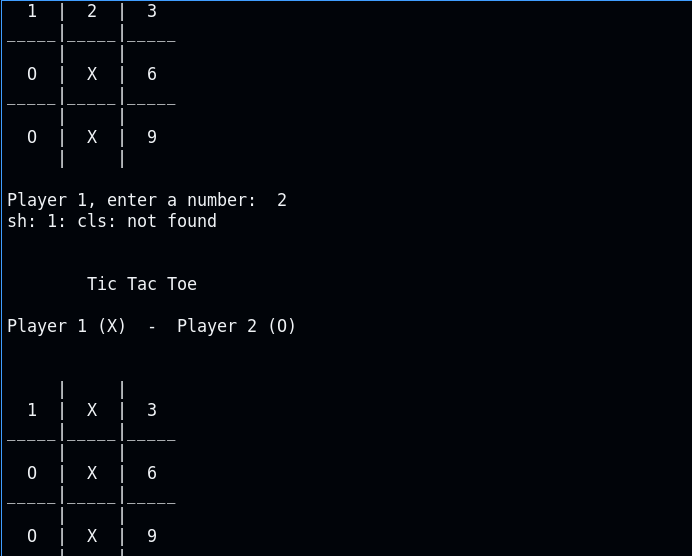Create a tic tac toe game using c++ that will allow two users to play that game. The program asks for moves alternately from player X and player O.

Hint: create two functions one from display the board and the other to check whether the game is over with a result of the game is in progress so that he/she will give input or the game is over with no result.

123=Win

456=Win

789=Win

147=Win

258=Win

369=Win

357=Win

159=WinHow do you make a Tic Tac Toe in C++?

#include <iostream>
using namespace std;

char square = {'o','1','2','3','4','5','6','7','8','9'};

int checkwin();
void board();

int main()
{
int player = 1,i,choice;

char mark;
do
{
board();
player=(player%2)?1:2;

cout << "Player " << player << ", enter a number: ";
cin >> choice;

mark=(player == 1) ? 'X' : 'O';

if (choice == 1 && square == '1')
{
square = mark;
}
else if (choice == 2 && square == '2')
{
square = mark;
}
else if (choice == 3 && square == '3')
{
square = mark;
}
else if (choice == 4 && square == '4')
{
square = mark;
}
else if (choice == 5 && square == '5')
{
square = mark;
}
else if (choice == 6 && square == '6')
{
square = mark;
}
else if (choice == 7 && square == '7')
{
square = mark;
}
else if (choice == 8 && square == '8')
{
square = mark;
}
else if (choice == 9 && square == '9')
{
square = mark;
}
else
{
cout<<"Invalid move ";

player--;
cin.ignore();
cin.get();
}
i=checkwin();

player++;
}while(i==-1);
board();
if(i==1)
{
cout<<"==>\aPlayer "<<--player<<" win ";
}
else
{
cout<<"==>\aGame draw";
}

cin.ignore();
cin.get();
return 0;
}

/*********************************************
FUNCTION TO RETURN GAME STATUS
1 FOR GAME IS OVER WITH RESULT EITHER PLAYER ONE WIN OR PLAYER TWO
-1 FOR GAME IS IN PROGRESS IF USER HAS INPUT INPUT ON INVALID PLACE
O GAME IS OVER WITH NO RESULT IT IS TIED
**********************************************/

int checkwin()
{
if (square == square && square == square)
{
return 1;
}
else if (square == square && square == square)
{
return 1;
}
else if (square == square && square == square)
{
return 1;
}
else if (square == square && square == square)
{
return 1;
}
else if (square == square && square == square)
{
return 1;
}
else if (square == square && square == square)
{
return 1;
}
else if (square == square && square == square)
{
return 1;
}
else if (square == square && square == square)
{
return 1;
}
else if (square != '1' && square != '2' && square != '3'
&& square != '4' && square != '5' && square != '6'
&& square != '7' && square != '8' && square != '9')
{
return 0;
}
else
{
return -1;
}
}

/******************************************************************************************************
FUNCTION TO DRAW BOARD OF TIC TAC TOE WITH PLAYERS MARK BY USING ABOVE SQUARE INITIALIZE VARIABLE
*******************************************************************************************************/

void board()
{
system("cls");
cout << "\n\n\tTic Tac Toe\n\n";

cout << "Player 1 (X) - Player 2 (O)" << endl << endl;
cout << endl;

cout << " | | " << endl;
cout << " " << square << " | " << square << " | " << square << endl;

cout << "_____|_____|_____" << endl;
cout << " | | " << endl;

cout << " " << square << " | " << square << " | " << square << endl;

cout << "_____|_____|_____" << endl;
cout << " | | " << endl;

cout << " " << square << " | " << square << " | " << square << endl;

cout << " | | " << endl << endl;
}